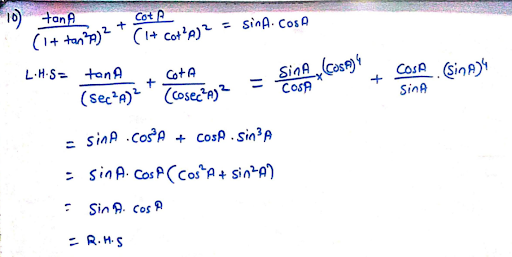# Prove That: Tan a ( 1 + Tan 2 a ) 2 + Cot a ( 1 + Cot 2 a ) 2 = Sin a Cos a

more_vert

Proove that : $$\frac{\tan A}{\left( 1 + \tan^2 A \right)^2} + \frac{\cot A}{\left( 1 + \cot^2 A \right)^2} = \sin A \cos A$$

more_vert

verifiedHence , prooved

## Related questions